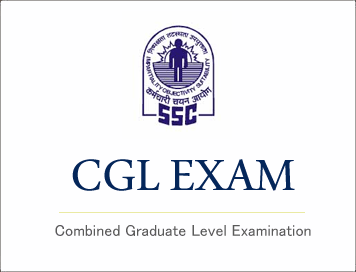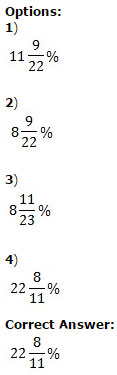# SSC CGL (Tier - 1) Online Exam Paper - 2016 "held on 10 September 2016" Evening Shift (Quantitative Aptitude)## SSC CGL (Tier - 1) Online Exam Paper - 2016 "held on 10 September 2016" Evening Shift (Quantitative Aptitude)

EXAM DATE : 10-September-2016
EXAM START TIME :
16:15:00
EXAM NAME :
SSC Examination 2016

Question 51.A and B undertake a piece of work for Rs. 250 . A alone can do that work in 5 days and B alone can do that work in 15 days . With the help of C, they finish the work in 3 days . If every one gets paid in proportion to work done by them, the amount C will get is

Options:

1) Rs.50
2) Rs.100
3) Rs.150
4) Rs.200

Question 52.Dealer purchased an article for Rs. 900 and fixes the list price in such a way that he gains 20% after allowing 10% discount, then the list price is

Options:

1) Rs.1180
2) Rs.1080
3) Rs.1200
4) Rs.1100

Question 53.Cost price of 28 articles is equal to Sale price of 21 articles, then percentage of profit is

Options:

1) 12%
2) 33⅓ %
3) 20%
4) 22%

Correct Answer: 33⅓ %

Question 54.In the last financial year, a car company sold 41,800 cars . In this year, the target is to sell 51,300 cars . By what percent must the sale be increased ?Question 55.If the speed of a boat in still water is 20 km/hr and the speed of the current is 5 km/hr, then the time taken by the boat to travel 100 km with the current is

Options:

1) 2 hrs
2) 3 hrs
3) 4 hrs
4) 7 hrs

Correct Answer: 4 hrs

Question 56.If a sum of money doubles itself in 8 yrs, then the interest rate in percentage is ?

Options:

1) 8 ½%
2) 10%
3) 10 ½ %
4) 12 ½ %

Correct Answer: 12 ½ %

## Study Kit for SSC CGL EXAM

Question 57.If ( x - 5)2 + (y - 2)2 + (z - 9)2 = 0 , then value of (x + y - z) is

Options:

1) 16
2) -1
3) -2
4) 12

Question 58.Options:

1) 2201
2) 2203
3) 2207
4) 2213

Question 59.Points P , Q and R are on a circle such that ∠PQR = 40° and ∠QRP = 60° . Then the subtended angle by arc QR at the centre is

Options:

1) 80°
2) 120°
3) 140°
4) 160°

Question 60.Question 61.If α + β = 90° and α:β = 2:1, then the ratio of cosα to cosβ is

Options:

1) 1:√3
2) 1:3
3) 1:√2
4) 1:2

Question 62.The least number to be added to 13851 to get a number which is divisible by 87 is

Options:

1) 18
2) 43
3) 54
4) 69

Question 63.Two numbers are in the ratio 3:5. If 6 is added to both of them, the ratio becomes 2:3. The numbers are

Options:

1) 21 and 35
2) 30 and 50
3) 24 and 40
4) 18 and 30

Correct Answer: 18 and 30

Question 64.The average weight of 10 parcels is 1.7 kg. Addition of another new parcel reduces the average weight by 60 gram. What is the weight , in kg, of the new parcel?

Options:

1) 1.04
2) 1.08
3) 1.4
4) 1.8

Question 65.Options:

1) 1000
2) 9000
3) 1
4) 9

Question 66.Options:

1) 1
2) 2
3) 3
4) 4

Question 67.In an isosceles triangle ΔABC, AB = AC and ∠A = 80°. The bisector of ∠B and ∠C meet at D. The ∠BDC is equal to

Options:

1) 90 o
2) 100 o
3) 130 o
4) 80 o

Correct Answer: 130 o

Question 68.The length of a chord which is at a distance of 12 cm from the centre of a circle of radius 13 cm i

Options:

1) 10 cm
2) 5 cm
3) 6 cm
4) 12 cm

Correct Answer: 10 cm

Question 69.If Ɵ is positive acute angle and 7cos2Ɵ + 3 sin2Ɵ = 4, then the value of θ is

Options:

1) 60 o
2) 30 o
3) 45 o
4) 90 o

Correct Answer: 60 o

Question 70.Radius of cross section of a solid right circular cylindrical rod is 3.2 dm. The rod is melted and 44 equal solid cubes of side 8 cm are formed. The length of the rod is (Take Π = 22/7)

Options:

1) 56 cm
2) 7 cm
3) 5.6 cm
4) 0.7 cm

Correct Answer: 7 cm

Question 71.The angles of elevation of the top of a tower from two points at a distance of 4 m and 9 m from the base of the tower and in the same straight line with it are complementary. The height of the tower is

Options:

1) 4 m
2) 7 m
3) 9 m
4) 6 m

Correct Answer: 6 m

Question 72.Study the histogram of marks (in Mathematics) distribution of 50 students of class IX and answer the following questions.The number of students who have secured marks less than 60 is

Options:

1) 12
2) 15
3) 33
4) 7

Question 73.Study the histogram of marks (in Mathematics) distribution of 50 students of class IX and answer the following questions.

The average marks of the students are

Options:

1) 53.2
2) 45.5
3) 60.2
4) 55.5

Question 74.Study the histogram of marks (in Mathematics) distribution of 50 students of class IX and answer the following questions.

The number of students who have scored between 39 and 80 is

Options:

1) 22
2) 18
3) 37
4) 15

Question 75.Study the histogram of marks (in Mathematics) distribution of 50 students of class IX and answer the following questions.

Percentage of students who have secured marks more than 59 is

Options:

1) 13
2) 17
3) 34
4) 26